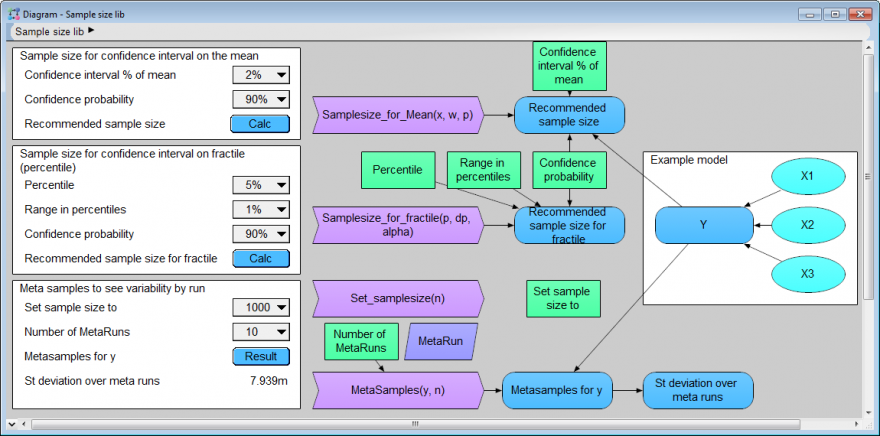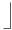# Selecting the Sample Size

(Redirected from Selecting a Sample Size)

Analytica represents the uncertain value (prob value) of an uncertain quantity as a random sample from its probability distribution -- an array of sample values indexed by Run. You can use the uncertainty view to show each uncertain quantity as a probability density function (PDF) , cumulative distribution function (CDF), selected statistics, fractiles (percentiles), or even the underlying random sample. By default, it uses a sample size of 1000. A larger sample size reduces random noise in the estimated distribution, increasing the accuracy of estimates of the mean, median, median, and other statistics. distribution. But the computation time and memory used go up roughly linearly with the sample size.

## Changing the sample size

You can change the sample size from its default of 1000 in the Uncertainty Setup dialog from the Result menu or just press control+U ("U" for uncertainty):

## Sample Size and Smooth Probability Distributions

When you are first building a model, it's usually best to start with a moderate sample size, such as the default 1000 -- or even smaller if you have a large model. That way, you can test it out as you build without having to wait for long calculation times. When you are happy with your model and ready to generate results for a client or report, you might then increase the sample size to provide greater accuracy.

How many samples do you need? It depends on what you want the results for. If you just want a rough idea of the range of key results, say 10th to 90th percentile, a sample of 100 to 1000 may be plenty. You can visualize distributions by selecting an Uncertainty views in the Result window. The cumulative probability distribution shows less noise -- i.e. roughness due to random sampling that does not reflect anything real about the actual distribution -- than the probability density function.

If you find the density view has too much noise in your initial view, you can reduce the noise -- without using a larger sample size --with the Smoothing option in the Probability density tab of the Uncertainty Setup dialog:

We generally recommend using the default smoothing factor. Higher levels of smoothing may give misleading results, e.g. with inappropriately wide tails.

## Function library for choosing a sample size

This Analytica library includes functions to estimate the sample size that you need to estimate the mean or a fractile (percentile) of a probability distribution with a specified confidence interval. It also contains a function to create a meta sample -- that is to rerun a Monte Carlo simulation multiple times if you want to estimate the variability of a statistic over multiple runs for a given sample size.The next sections explain the rationale and statistics underlying the methods in this model.

### Convergence and statistics

Even experienced risk analysts sometimes resort to "convergence" testing to decide on sample size: They run simulations with increasing sample sizes to see "when the results seem to converge to a consistent value." Or they compare multiple runs to see how well they agree. This kind of empirical exploration of sample size can be very time consuming. But, it is usually unnecessary.

The key point is that the results generated by Monte Carlo simulation are a random sample from the "true" distribution, assuming all the input distributions are well-chosen. This means that you can use simple statistics to select the sample size you need provided you can specify well-defined goals -- for example, if you want to estimate the true mean of the distribution has a 95% probability of being within 1% of the estimated mean -- or if you want to know that the estimated median (50th percentile) has a 95% probability of being between the estimated 49th and 51st percentile. Below we show how to estimate the sample sizes needed to obtain results with the specified accuracy. You can also download an Analytica library with functions to help you do these calculations.

Note that these results assume you are using simple Monte Carlo simulation. Analytica also offers median and random Latin hypercube sampling as other options in the Uncertainty Setup dialog. These often converge a little faster than Monte Carlo -- i.e. they provide more accurate results for a given sample size. But, you can use the same statistical methods to estimate needed sample size, and be confident that they will be adequate whichever sample method you use.

### Estimating sample size for a confidence interval on the Mean

First, suppose you are interested in the precision of the mean of a result variable «y» that your client cares about. Suppose you have a random sample of «m» values from «y» generated by Monte Carlo simulation:

$\displaystyle{ (y_1, y_2, y_3, …y_m) }$
(1)

Here's are the standard estimates for the mean and standard deviation of «y»:

$\displaystyle{ \vec y=\sum_{i=1}^m \frac { y_i }m }$
(2)
$\displaystyle{ s^2=\sum_{i=1}^m \frac { (y_i - \vec y)^2} {m - 1} }$
(3)

The Central Limit Theorem says that the sampling distribution on the mean tends to normal for large «m» for any distribution for «y» (if it has finite variance). Given «c» is the deviation for the unit normal enclosing probability «α», this gives us the confidence interval on the true mean with probability «α»:

$\displaystyle{ \left (\vec y-c\frac {s} {\sqrt m}, \vec y + c\frac {s} {\sqrt m}\right ) }$
(4)

What sample size do you need to estimate the mean of «y» with an «α» confidence interval smaller than «w» units wide? You need to make sure that:

$\displaystyle{ w\gt 2c \frac {s}{\sqrt m} }$
(5)

Rearranging the inequality:

$\displaystyle{ m\gt \left ( \frac {2cs}{w}\right ) ^2 }$
(6)

To use this, first make a small Monte Carlo run with, say, 10 values to get an initial estimate of the variance of «y» — that is, «s2». You can then use equation (6) to estimate how many samples reduce the confidence interval to the requisite width «w».

For example, suppose you want a 95% confidence interval for the mean that is less than 20 units wide. Suppose your initial sample of 10 gives s = 40. The deviation «c» enclosing a probability of 95% for a unit normal is about 2. Substituting these numbers into equation (6), we get:

$\displaystyle{ m\gt \left ( \frac {2 \times 2 \times 40}{20} \right )^2 = 8^2 = 64 }$
(7)

So, to get the desired precision for the mean, you should set the sample size to about 64.

### Estimating Confidence Intervals for Fractiles

Another way to select a sample size is to obtain a confidence interval for the median or other fractile (percentile) of a the probability distribution for an uncertain result of interest. Suppose that we label the sample «m» values of «y» so that they are in increasing order:

$\displaystyle{ y_1 \le y_2 \le ...y_m }$

Define «c» as the deviation enclosing probability «α» of the unit normal (c := -CumNormalInv((1-alpha)/2)). Then these two sample values enclose the confidence interval on the pth percentile «Yp»:

$\displaystyle{ (y_i, y_k) }$

where:

$\displaystyle{ i = \left \lfloor mp - c \sqrt {mp (1-p)} \right \rfloor }$
(8)

$\displaystyle{ k = \left \lceil mp + c \sqrt {mp (1-p)} \right \rceil }$
(9)
Note
The brackets in equations (8) and (9) above mean round upand round down, since they are computing numbers that need to be integers.

Suppose you want to achieve sufficient precision such that the «$\displaystyle{ \alpha }$» confidence interval for the pth fractile «Yp» is given by (y1, y2), where «yi» is an estimate of $\displaystyle{ {Y_p-{_\Delta}{_p}} }$, and «yk» is an estimate of $\displaystyle{ {Y_p+{_\Delta}{_p}} }$. In other words, you want «$\displaystyle{ \alpha }$» confidence of «Yp» being between the sample values used as estimates of the ($\displaystyle{ p-{_\Delta}{_p}) }$) and ($\displaystyle{ p+{_\Delta}{_p}) }$) fractiles. What sample size do you need? Ignoring the rounding, you have approximately:

$\displaystyle{ i = m(p-{\Delta}p), k = m(p+{\Delta} p) }$
(10)

Thus:

$\displaystyle{ k - i = 2m{\Delta}p }$
(11)

From equations (8) and (9) above, you have:

$\displaystyle{ k - i = 2c\sqrt {mp(1-p)} }$
(12)

Equating the two expressions for k - 1 , you obtain:

$\displaystyle{ 2mp\Delta p = 2c \sqrt {mp(1-p)} }$
(13)

$\displaystyle{ m=p(1- p) \left (\frac {c}{\Delta p} \right )^2 }$
(14)

For example, suppose you want to be 95% confident that the estimated fractile Y.90 is between the estimated fractiles Y.85 and Y.95. So you have $\displaystyle{ \Delta p }$ = 0.05, and c ≈ 2. Substituting the numbers into equation (14), you get:

$\displaystyle{ m = 0.90 \times (1 - 0.90) \times \left (\frac {2}{0.05} \right )^2 = 144 }$
(15)

On the other hand, suppose you want the credible interval for the least precise estimated percentile (the 50th percentile) to have a 95% confidence interval of plus or minus one estimated percentile. Then:

$\displaystyle{ m = 0.5 \times (1 - 0.5) \times \left (\frac {2}{0.01} \right )^2 = 10,000 }$
(16)

These results are completely independent of the shape of the distribution. If you find this an appropriate way to state your requirements for the precision of the estimated distribution, you can determine the sample size before doing any runs to see what sort of distribution it might be.